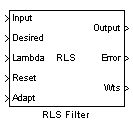# RLS Filter

Compute filtered output, filter error, and filter weights for given input and desired signal using RLS adaptive filter algorithm

## Library

`dspadpt3`

•## Description

The RLS Filter block recursively computes the least squares estimate (RLS) of the FIR filter weights. The block estimates the filter weights, or coefficients, needed to convert the input signal into the desired signal. Connect the signal you want to filter to the Input port. The input signal can be a scalar or a column vector. Connect the signal you want to model to the Desired port. The desired signal must have the same data type, complexity, and dimensions as the input signal. The Output port outputs the filtered input signal. The Error port outputs the result of subtracting the output signal from the desired signal.

The corresponding RLS filter is expressed in matrix form as

`$\begin{array}{l}k\left(n\right)=\frac{{\lambda }^{-1}P\left(n-1\right)u\left(n\right)}{1+{\lambda }^{-1}{u}^{H}\left(n\right)P\left(n-1\right)u\left(n\right)}\hfill \\ y\left(n\right)=w\left(n-1\right)u\left(n\right)\hfill \\ e\left(n\right)=d\left(n\right)-y\left(n\right)\hfill \\ w\left(n\right)=w\left(n-1\right)+{k}^{H}\left(n\right)e\left(n\right)\hfill \\ P\left(n\right)={\lambda }^{-1}P\left(n-1\right)-{\lambda }^{-1}k\left(n\right){u}^{H}\left(n\right)P\left(n-1\right)\hfill \end{array}$`

where λ-1 denotes the reciprocal of the exponential weighting factor. The variables are as follows

VariableDescription

n

The current time index

u(n)

The vector of buffered input samples at step n

P(n)

The inverse covariance matrix at step n

k(n)

The gain vector at step n

$w\left(n\right)$

The vector of filter-tap estimates at step n

y(n)

The filtered output at step n

e(n)

The estimation error at step n

d(n)

The desired response at step n

λ

The forgetting factor

The implementation of the algorithm in the block is optimized by exploiting the symmetry of the inverse covariance matrix P(n). This decreases the total number of computations by a factor of two.

Use the Filter length parameter to specify the length of the filter weights vector.

The Forgetting factor (0 to 1) parameter corresponds to λ in the equations. It specifies how quickly the filter “forgets” past sample information. Setting λ=`1` specifies an infinite memory. Typically, $1-\frac{1}{2L}<\lambda <1$, where L is the filter length. You can specify a forgetting factor using the input port, Lambda, or enter a value in the Forgetting factor (0 to 1) parameter in the Block Parameters: RLS Filter dialog box.

Enter the initial filter weights, $\stackrel{^}{w}\left(0\right)$, as a vector or a scalar for the Initial value of filter weights parameter. When you enter a scalar, the block uses the scalar value to create a vector of filter weights. This vector has length equal to the filter length and all of its values are equal to the scalar value.

The initial value of P(n) is

`$\frac{1}{{\sigma }^{2}}I$`

where you specify ${\sigma }^{2}$ in the Initial input variance estimate parameter.

When you select the Adapt port check box, an Adapt port appears on the block. When the input to this port is nonzero, the block continuously updates the filter weights. When the input to this port is zero, the filter weights remain at their current values.

When you want to reset the value of the filter weights to their initial values, use the Reset input parameter. The block resets the filter weights whenever a reset event is detected at the Reset port. The reset signal rate must be the same rate as the data signal input.

From the Reset input list, select `None` to disable the Reset port. To enable the Reset port, select one of the following from the Reset input list:

• `Rising edge` — Triggers a reset operation when the Reset input does one of the following:

• Rises from a negative value to a positive value or zero

• Rises from zero to a positive value, where the rise is not a continuation of a rise from a negative value to zero; see the following figure• `Falling edge` — Triggers a reset operation when the Reset input does one of the following:

• Falls from a positive value to a negative value or zero

• Falls from zero to a negative value, where the fall is not a continuation of a fall from a positive value to zero; see the following figure• `Either edge` — Triggers a reset operation when the Reset input is a `Rising edge` or `Falling edge`, as described above

• `Non-zero sample` — Triggers a reset operation at each sample time that the Reset input is not zero

Select the Output filter weights check box to create a Wts port on the block. For each iteration, the block outputs the current updated filter weights from this port.

## Examples

The `rlsdemo` example illustrates a noise cancellation system built around the RLS Filter block.

## Parameters

Filter length

Enter the length of the FIR filter weights vector.

Specify forgetting factor via

Select `Dialog` to enter a value for the forgetting factor in the Block parameters: RLS Filter dialog box. Select `Input port` to specify the forgetting factor using the Lambda input port.

Forgetting factor (0 to 1)

Enter the exponential weighting factor in the range ```0 ```≤λ≤`1`. A value of `1` specifies an infinite memory. Tunable (Simulink).

Initial value of filter weights

Specify the initial values of the FIR filter weights.

Initial input variance estimate

The initial value of 1/P(n).

Select this check box to enable the Adapt input port.

Reset input

Select this check box to enable the Reset input port.

Output filter weights

Select this check box to export the filter weights from the Wts port.

## References

Hayes, M.H. Statistical Digital Signal Processing and Modeling. New York: John Wiley & Sons, 1996.

## Supported Data Types

• Double-precision floating point

• Single-precision floating point

 Kalman Adaptive Filter (Obsolete) DSP System Toolbox LMS Filter DSP System Toolbox Block LMS Filter DSP System Toolbox Fast Block LMS Filter DSP System Toolbox

See Noise Cancellation in Simulink Using Normalized LMS Adaptive Filter for related information.

Watch now